# Permutation and Combination - Introduction and Examples (Part - 1) Notes | Study Quantitative Aptitude for Competitive Examinations - Quant

## Quant: Permutation and Combination - Introduction and Examples (Part - 1) Notes | Study Quantitative Aptitude for Competitive Examinations - Quant

The document Permutation and Combination - Introduction and Examples (Part - 1) Notes | Study Quantitative Aptitude for Competitive Examinations - Quant is a part of the Quant Course Quantitative Aptitude for Competitive Examinations.
All you need of Quant at this link: Quant

Permutation

Permutation is basically called as a arrangement where order does matters.Here we need to arrange the digits , numbers , alphabets, colors and letters taking some or all at a time.It is represented as nPr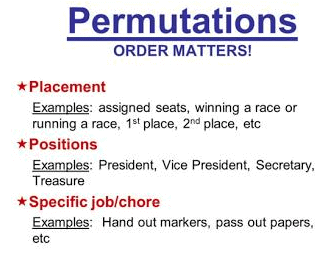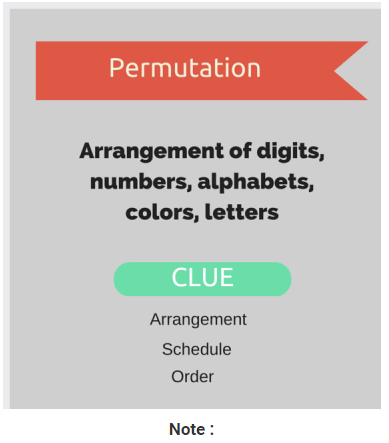1. nPr = n! / (n-r)!

2. If from the total set of n numbers p is of one kind and q ,r are others respectively then nPr = n! / p! × q! × r!.

3. nPn = n!

Combination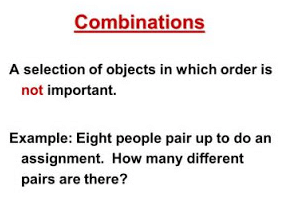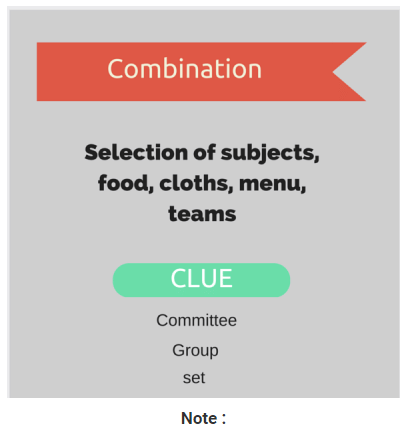1.  nCr = n!/ r! × (n-r)!
2.  nC0 = 1
3.  nCn = 1
4.  nCr =  nCn - r
5.  nCa =  nCb => a = b => a+b = n
6. nC0nC1nC2nC3+ ...............+ nC= 2n

Permutation vs Combination

In both the things main difference is of order .In permutation order matters while in combination it does not.

Basic Difference :

1. order
2. arrange or choose
3. number of permutation > number of combination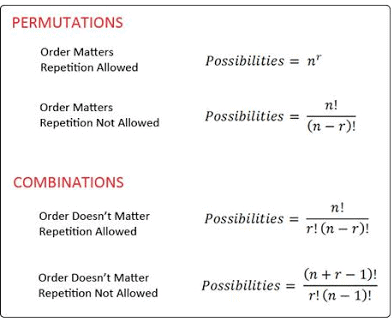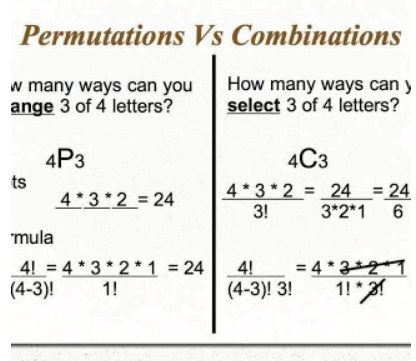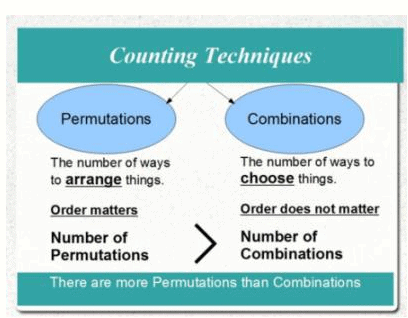In Arrangements we have,

Total no. of arrangements = total no. of groups or selection × r!

where r is the no. of objects in each group or selection. So nPrnCr  × r!

Questions :

1. How many triangles can be formed with four points (A,B,C & D) in a plane ? It is given that no three points are col-linear(not comes in straight line).From the three points A,B and C have only one triangle with these points.

Sol:

Here in this question  , Order of digit does not matter so it is a combination.

nCr = n!/ r! × (n-r)!

4C= 4!/3! × ( 4- 3)!

4C= 4!/3! × 1!

4C= 4!/3! × 1!

4C= 4

Or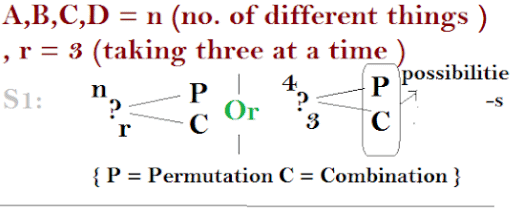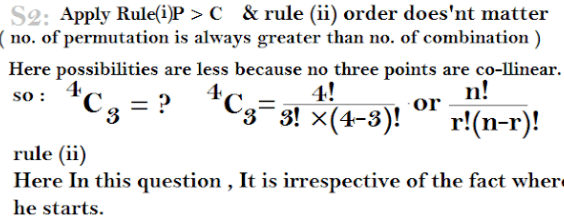2. How many number plates of 3 digit can be formed with four digits 1,2,3 and 4 ?

Sol:

Here, the order of arrangement of digits does matter.

nPr = n! / (n-r)!

nPr = 4! / (4-3)!

4P3 = 4! / 1!

4P3 = 4!

4P3 = 24

Factorial Notation

To solve problem like this you must have the knowledge of factorial.Factorial is represented as like " ! ".The Factorial notation is :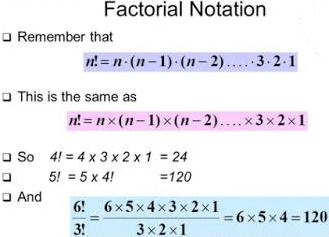The document Permutation and Combination - Introduction and Examples (Part - 1) Notes | Study Quantitative Aptitude for Competitive Examinations - Quant is a part of the Quant Course Quantitative Aptitude for Competitive Examinations.
All you need of Quant at this link: QuantUse Code STAYHOME200 and get INR 200 additional OFF

## Quantitative Aptitude for Competitive Examinations

33 videos|34 docs|143 tests

Track your progress, build streaks, highlight & save important lessons and more!

,

,

,

,

,

,

,

,

,

,

,

,

,

,

,

,

,

,

,

,

,

;